﻿ 6.3 The Zero Coupon Bond Caseoffice (412) 9679367
fax (412) 967-5958
toll-free 1 (800) 214-3480

6.3 The Zero Coupon Bond Case

 I

n the cash matching section, you saw that you can control all interest rate risk perfectly if you construct the exact synthetic equivalent of the underlying position. The problem with this approach is that large positions imply tens of thousands of different cash flow points over time. It is unlikely that the markets exist to create a synthetic equivalent. Even if sufficient markets were available, the transaction cost associated with creating such a security would be onerous.

This leads us to a question: Do simpler "solutions" exist that would allow a trader to manage the exposure of a position to interest rate risk using fewer securities?

Investment Example

In this example, we will consider the impact of large yield curve shifts upon a small position. Consider the following position, which generates cash flows at the end of each year for four years. The cash flows associated with each component are described by the following set of timelines:

Security Units

Security I -14

Cash in 1-year money market line \$5,300

2-Year zero-coupon bond 51

3-Year zero-coupon bond 0

The following timelines describe the cash flow from each security.

Timeline for Per Share Cash Flows from Security I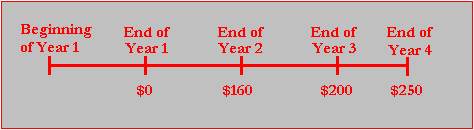Timeline for Cash Flows from 1-Year Money Market Line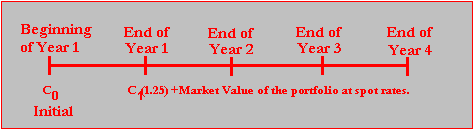Timeline for Cash Flows from 2-Year Zero-Coupon Bond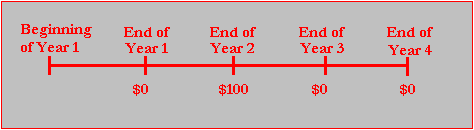Timeline for Cash Flows from 3-Year Zero-Coupon Bond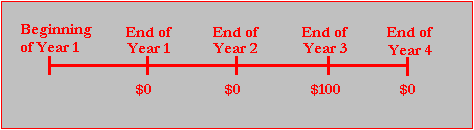Online, you can work with this problem using the interactive online calculator below.  You can click on the cell besdie Security 1 under Value (307.20) to bring up the exposure profile for the coupon bond.:

To access the The default dataset is the problem at hand. By clicking on the Cash Flows button (beside Numeric) you can see the cash flows associated with the four securities. The fifth "security" is \$100 cash at time zero.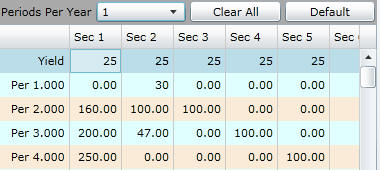Click on Done and then Enter the position as follows:

Security Units

Security I -14

Cash in 1-year money market (Security 5) \$5,300

2-Year zero-coupon bond (Security 3) 51

3-Year zero-coupon bond (Security 4) 0

Note:  You enter a position directly online by double clicking on the appropriate cell under Units, and typing the number directly into the cell followed by Return or Enter.  If you make an error you can use the delete or backspace keys.

Your entered position should appear as follows:

Note:  Be sure to click beside Portfolio and then click on OK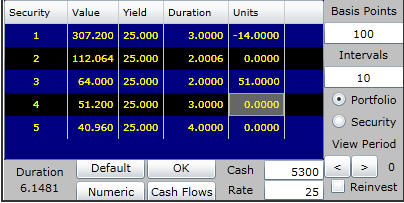The exposure of this position to interest rate risk appears as follows: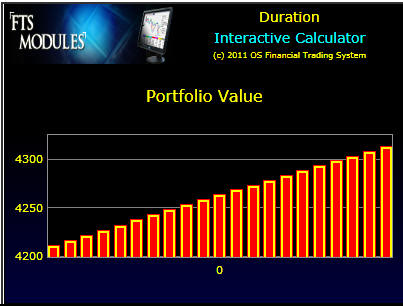Online above you can also specify the size of the initial shift in the yield curve (in basis points). In the following screen, a total shift of +/- 2000 basis points (20%) with 2 partitions either side of the current 25% is specified:

Online Enter under Basis Points 2000 and Intervals 10 then click beside Portfolio and finally click OK.  This gives a finer plot of the interest rate bucket shifts.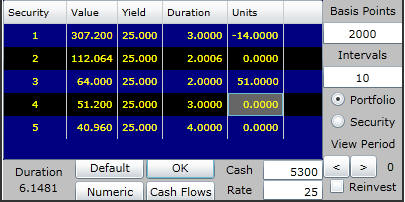Online, you can see, this position benefits from an increase in interest rates and is hurt by a decrease.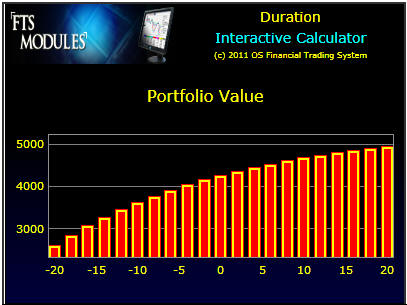Numerically, the value of these two securities can be viewed by clicking on the Numeric button: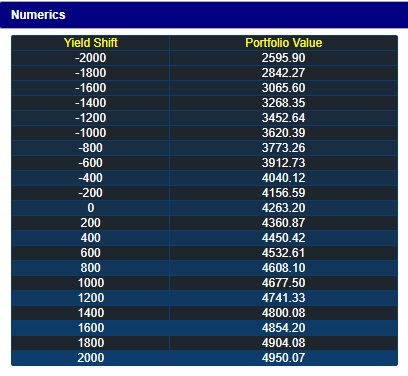The origin is the flat yield curve of 25% and the shifts are +/1 10% and 20%. You can see that the present value of the portfolio is exposed to shifts in the yield curve.

For example, let the current spot yield curve be flat and equal to 25% for Years 1- 4. If the yield curve remains at 25%, then the current value of this position is:

-\$1,036.80 + \$5,300 = \$4,263.20

From the above you can see that Cash = \$5300 plus long positions (assets) = 3264.00 and short positions (liabilities) = 4300.80. The aggregate value is \$5300 + \$3264.00 - \$4300.80 = \$4,263.20.

In practice, we must consider the effects of a yield curve shift at the end of some period of time. That is, the position is invested at the current spot rate and then during the period the yield curve shifts to a new level. To consider the effects of this scenario, compute the value of this position at the end of the year:

\$4,263.20 * 1.25 = \$5,329

Online, you can verify this by computing the future value of cash flows at the end of Year 1 assuming 25%, 25%, 25%. The end of year value of this position when there is no change in the yield curve is obtained by moving View Period forward 1-period (as illustrated below) and checking the checkbox "Reinvest.":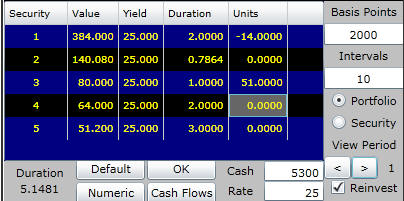Numerically, \$5329 = \$5300*1.25 + \$4080.00 - \$5376.00.

\$5,329 = \$5,300 * 1.25 - \$1,296

To see this click on the Numeric button and view the 0 Yield Shift.Now, suppose the yield curve shifts up during the first year so that at year end it is a flat 35%. This shift reduces the value of both the asset (i.e., the long position) and the liability (i.e., the short position). Overall, the total value increases to:

\$5,300*1.25 - \$840.38 = \$5,784.62

Which you can see above in the Portfolio Values window beside 10.00 (i.e., shift up by 10% in yield) and View Period 1 and click on the Numeric button:You can verify for other realizations in the same way that the end of year position value equals:

Yield Curve at end of Year 1: Value of Position

(1-, 2-, and 3-year spot rate) at end of Year 1

5%, 5%, 5% \$3785.69

15%,15%,15% \$4693.45

25%,25%,25% \$5329

35%,35%,35% \$5784.62

45%,45%,45% \$6117.61

That is, this position (when invested at the current spot rates) benefits from an increase in interest rates but is hurt by a decrease in interest rates.

Hedging Interest Rate Risk

How can you ensure that this position never falls below \$5,000?

An easy solution is to cash out. At the current yield curve this locks you into \$5,329, which satisfies your constraint with a little excess to spare. However, at any point in time, a firm's position consists of two types of short (liabilities) and long (assets) positions: those that are held for trading purposes and those that are not intended to be liquidated during the firm's normal course of business over the current period.

Suppose the liability, -14 units of Security I, cannot be traded but you still want to manage the interest rate risk associated with this position. In particular, you want to ensure that by year end its value does not fall below \$5,000 over the given range of yield curve shifts.

How can you achieve this?

Consider buying 84 3-year zeroes and selling 51 2-year zeroes.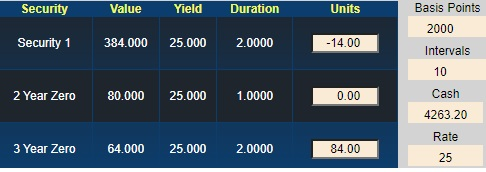Online, a very different picture emerges. The present value of this position appears as follows: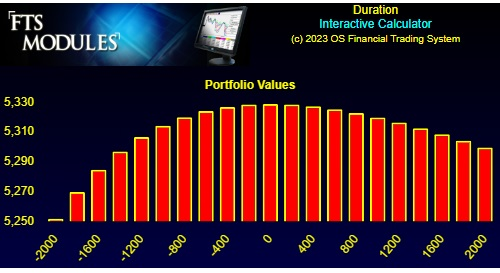Numerically, this appears as above. Note that in the above you need to enter your final position. That is, you sold 51 units at \$80 and bought 84 units at \$64.00. As a result, at time 0 you only have \$4263.20 cash to invest because 84 units at \$64 costs more than the revenue generated from selling 51 units at \$80.

Click on the Numeric button to see the range of fluctuations in actual position value by first scrolling to View Period 1.00 as below: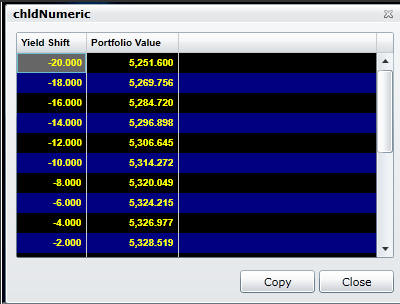In other words our position is now staying above \$5000 at year end, for a wide range of yield curve fluctuations! That is, your position is hedged against fluctuations in the yield curve.

In summary to hedge this position recall that you engaged in the following transactions at the beginning of the year:

i. Sold 51 units of Security 3 at \$64 (the present value of \$100 at the end of Year 2 using the spot flat 25% yield curve). This equals \$3,264 (51*\$64).

ii. Bought 84 units of Security 4 at \$51.20 (the present value of \$100 at the end of Year 3 using the spot flat 25% yield curve). This equals \$4,300.80 (84*\$51.20).

iii. Invested the remaining balance of cash in the money market at 25%. This equals \$4,263.20*1.25 (\$5,300 + \$3,264 - \$4,300.80 = \$4,263.20).

Numerically, the year-end value of this position, if there is no shift in the yield curve, equals:

\$4,263.20 * 1.25 - 14 * \$384 + 84 * \$64 = \$5,329

Yield Curve at end of Year 1: Value of Position

(1, 2, and 3 year spot rate) at End of Year 1

5%, 5%, 5% \$5251.60 (= \$5,329 - \$77.40)

15%,15%,15% \$5314.27 (= \$5,329 - \$14.73)

25%,25%,25% \$5329

35%,35%,35% \$5319.90 (= \$5,329 - \$9.10)

45%,45%,45% \$5299.61 (= \$5,299.61 - \$29.39)

You can see that this new position satisfies the \$5,000 constraint over a range of 2,000 basis points shift in the yield curve.

In this trading approach to hedging interest rate risk be sure to distinguish between the time of adjusting the position (the beginning of the year) with the time for which protection is desired (at the end of the year).

You may be wondering whether some general principle underlies this example. That is, what guided the choice of selling 51 units of Security 2 and buying 84 units of Security 3?

It turns out that a general principle does exist. This example was constructed by applying an important theorem, the bond immunization theorem. We develop this theory in the next topic, Macaulay's Duration, and then return to this example in topic 6.5 to see how it applies.